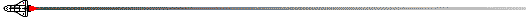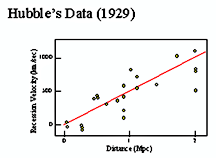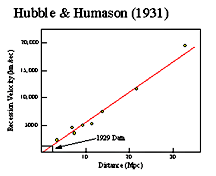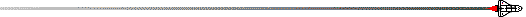# Redshift and Hubble's LawFor very far objects (beyond about 1 billion light-years) none of the above methods work. Scientists must move from direct observation to using observations in conjunction with a theory. The theory used to determine these very great distances in the universe is based on the discovery by Edwin Hubble that the universe is expanding.In 1929, Edwin Hubble announced that almost all galaxies appeared to be moving away from us. In fact, he found that the universe was expanding - with all of the galaxies moving away from each other. This phenomenon was observed as a redshift of a galaxy's spectrum. This redshift appeared to be larger for faint, presumably further, galaxies. Hence, the farther a galaxy, the faster it is receding from Earth. You can see this trend in Hubble's data shown in the images above. The velocity of a galaxy could be expressed mathematically as

v = H x d

where v is the galaxy's radial outward velocity, d is the galaxy's distance from Earth, and H is the constant of proportionality called the Hubble constant.

The exact value of the Hubble constant is still somewhat uncertain, but is generally believed to be around 65 kilometers per second for every megaparsec in distance. (A megaparsec is given by 1 Mpc = 3 x 106 light-years). This means that a galaxy 1 megaparsec away will be moving away from us at a speed of 65 km/sec, while another galaxy 100 megaparsecs away will be receding at 100 times this speed. So essentially, the Hubble constant reflects the rate at which the universe is expanding.

So to determine an object's distance, we only need to know its velocity. Velocity is measurable thanks to the Doppler shift. By taking the spectrum of a distant object, such as a galaxy, astronomers can see a shift in the lines of its spectrum and from this shift determine its velocity. Putting this velocity into the Hubble equation, they determine the distance. Note that this method of determining distances is based on observation (the shift in the spectrum) and on a theory (Hubble's Law). If the theory is not correct, the distances determined in this way are all nonsense. Most astronomers believe that Hubble's Law does, however, hold true for a large range of distances in the universe.

It should be noted that, on very large scales, Einstein's theory predicts departures from a strictly linear Hubble law. The amount of departure, and the type, depends on the value of the total mass of the universe. In this way a plot of recession velocity (or redshift) vs. distance, which is a straight line at small distances, can tell us about the total amount of matter in the universe and may provide crucial information about the mysterious dark matter.The StarChild site is a service of the High Energy Astrophysics Science Archive Research Center (HEASARC), Dr. Alan Smale (Director), within the Astrophysics Science Division (ASD) at NASA/ GSFC.

StarChild Authors: The StarChild Team
StarChild Graphics & Music: Acknowledgments
StarChild Project Leader: Dr. Laura A. Whitlock
Curator: J.D. Myers
Responsible NASA Official: Phil Newman